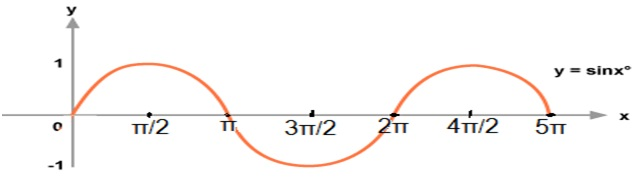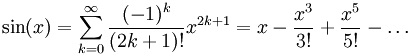# C program for sin(x) series

Sin x is a series of sin function of trigonometry; it can expand up to infinite number of term. Through this series, we can find out value of sin x at any radian value of sin x graph.
Submitted by Ashish Varshney, on March 19, 2018Reference: The Infinite Series Module.

## Sin x Series:Logic:

1. First the computer reads the value of x and limit from the user.
2. Now convert x to radian value x=x*(3.1415\180)
3. Then using for loop the value of sin(x) is calculated.
4. Finally the value of sin(x) is printed.

## Program for sin(x) series in C

```#include <stdio.h>
#include <math.h>

int fac(int x)
{
int i,fac=1;
for(i=1;i<=x;i++)
fac=fac*i;
return fac;
}

int main()
{
float x,Q,sum=0;
int i,j,limit;

printf("Enter the value of x of sinx series: ");
scanf("%f",&x);

printf("Enter the limit upto which you want to expand the series: ");
scanf("%d",&limit);

Q=x;
x = x*(3.1415/180);

for(i=1,j=1;i<=limit;i++,j=j+2)
{
if(i%2!=0)
{
sum=sum+pow(x,j)/fac(j);
}
else
sum=sum-pow(x,j)/fac(j);
}

printf("Sin(%0.1f): %f",Q,sum);
return 0;
}
```

Output

```Enter the value of x of sinx series: 40
Enter the limit upto which you want to expand the series: 5
Sin(40.0): 0.642772
```